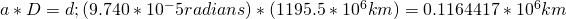# Calculating Diameter by Angular Diameter

Question:
My name is Eva and I was wondering if you would be willing or able to explain to me the
a x r = S equation? I am on one of my labs and the problems involve the distances of planets from Earth a (radians), r (10 to the 6th power), and S (10 to the 6th power). So far for Jupiter I’ve figured out that a = 4.693e-3 and r = 588.5, but I am confused how to find S. For Saturn the equation was already done and read: 9.740e-5 for a, 1195.5 for r, and 0.1164 for S. How was this answer found?

Okay.  So after a little investigation as to what exactly was meant by the question here, it relates to the calculation of diameter of an object in relation to the distance from the observer to the object and the angular size of the object at that distance.

a = angular size in radians
r = should actually be D for distance in kilometers
S = should be d for diameter of the object in kilometers

The labels make little difference if you understand how they are to be used.  I think that’s the biggest hurdle in this problem.  See diagram for graphical representation.

Now, the information that we have is as follows:

a = 9.740e-5 radians is roughly the angular size of Saturn viewed from Earth.
D = 1195.5e6 km is roughly the distance to Saturn.
d = what we’re searching for.

So, by our formula: a * D = d (or a x r = S if you like),So the diameter of Saturn, based on our calculations using the angular size and distance to Saturn, is about 116,441 km.